# Equations

Parent topic:
Algebra
•### Graph the Line

Activity

Steve Phelps

•### Graphing Linear Equations: Formative Assessment Items

Book

Tim Brzezinski

•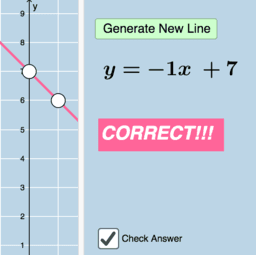### Quiz: Graphing Linear Equations (V3)

Activity

Tim Brzezinski

•### Solving Linear Systems by Graphing: Quiz (V1)

Activity

Tim Brzezinski

•### Linear Equation Generator

Activity

Tim Brzezinski

•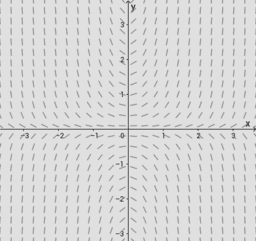### UQ-Applied Mathematical Analysis

Book

Juan Carlos Ponce Campuzano

•### Differential Equations

Book

Erik Jacobsen

•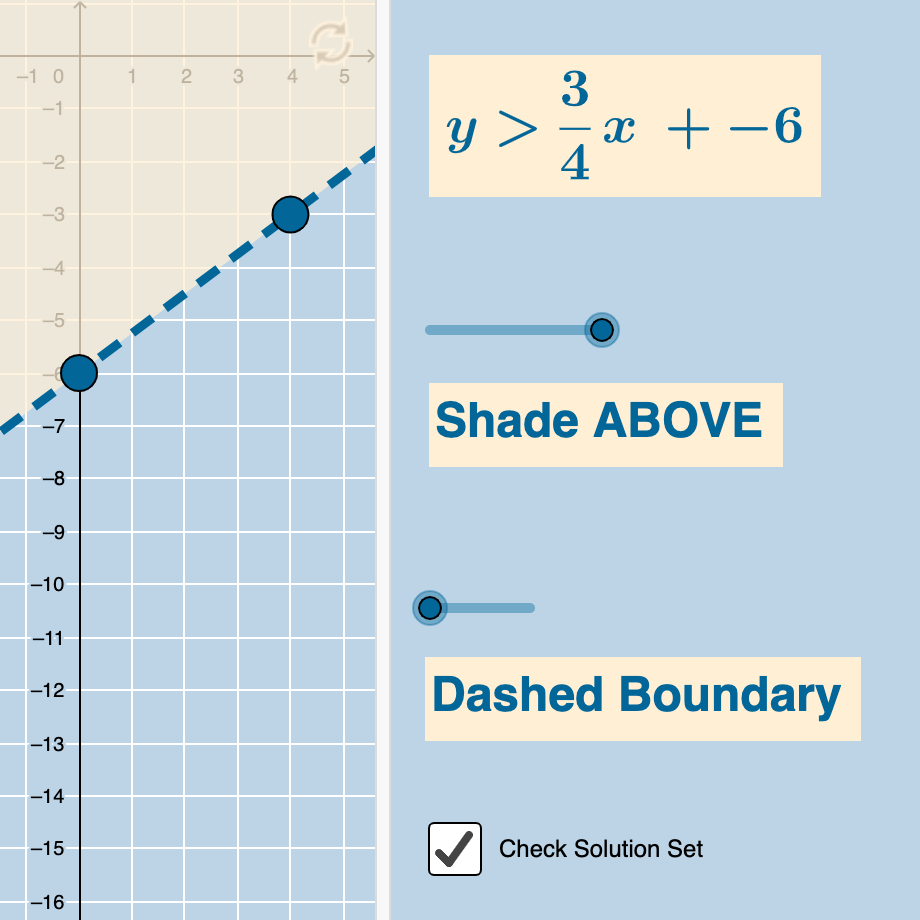### Graphing Linear Inequalities with 2 Variables (Quiz)

Activity

Tim Brzezinski

•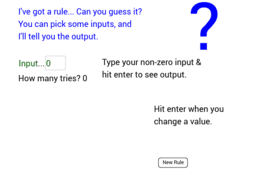### Guess My Rule (2013)

Activity

John Golden

•### Quiz: Graphing Linear Equations (V1)

Activity

Tim Brzezinski

•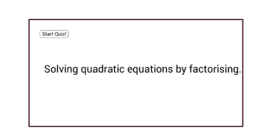Activity

Sparky

•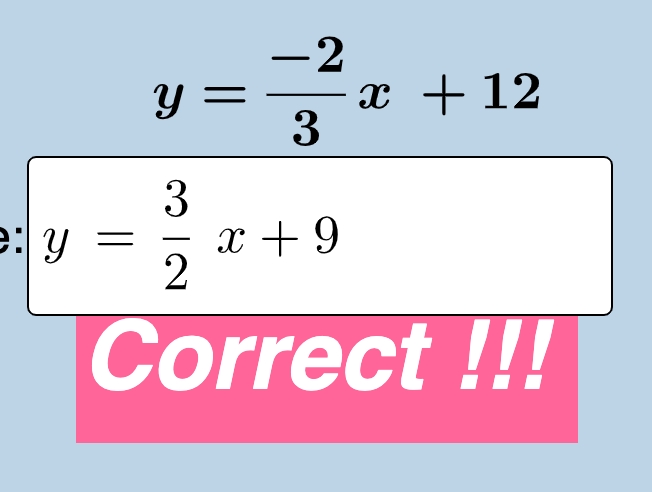### Parallel & Perpendicular (Writing Equations of Lines)

Activity

Tim Brzezinski

•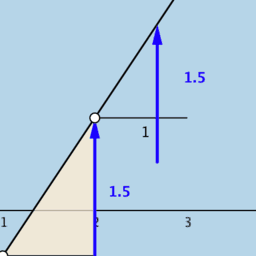### Equation of a Line: Dynamic Illustrator

Activity

Tim Brzezinski

•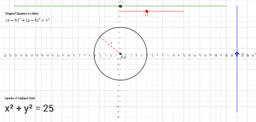### Equation & Graph of a Circle

Activity

Tim Brzezinski

•### Solving Linear Systems by Graphing: Quiz (V2)

Activity

Tim Brzezinski

•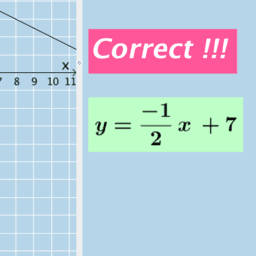### Writing the Equation of a Line (I-VA)

Activity

Tim Brzezinski

•### Parametric Equations Grapher

Activity

Tim Brzezinski

•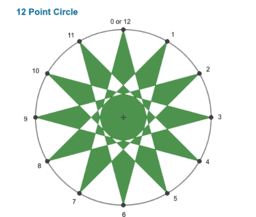### Apps for Formative Assessment Grade 10

Book

Lee W Fisher

•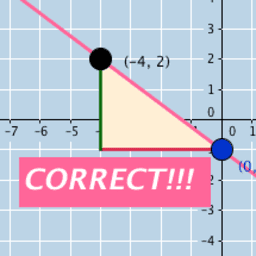### Quiz: Graphing Linear Equations (V2)

Activity

Tim Brzezinski

•### Graphing Linear Equations in 2 Unknowns

Activity

Anthony OR 柯志明

•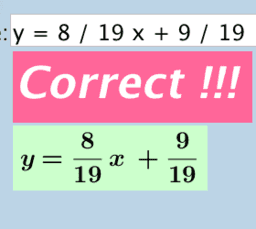### Standard Form to Slope-Intercept Form (Rewriting Linear Equations)

Activity

Tim Brzezinski

•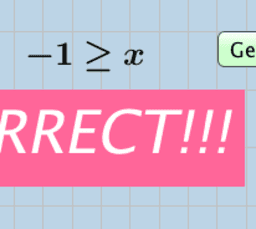### Graphing Basic Inequalities: 1 Variable (Quiz)

Activity

Tim Brzezinski

•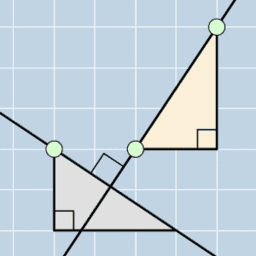### Coordinate & Analytic Geometry (90+)

Book

Tim Brzezinski

•### Graphing Linear Equations Written in Standard Form: Quiz (V1)

Activity

Tim Brzezinski